## 26 August 2017

### Answer key to UCEED 2017 Previous paper

You are here >> UCEED 2017 solution

You may download UCEED 2017 question paper here

### Answers for UCEED 2017 Exam paper

1) 12

Out of the all 6 surfaces of the given cube, it is given that three surfaces are green while the rest three are orange.
We are interested in finding the edges which have contact with one orange and one green surfaces,

The following image shows for one corner (top). As shown, although it has four small cubes at the top, the two edge cubes on either side will have two surfaces having a single color and another surface having another color, so they shouldn't be counted. So, for one edge, we have 2 cubes.

Now, the trick is to count the no. of such corners possible. Below figure shows all possible corners that lead to the required. All the invisible surfaces in the below image are green color.

As can be seen, we have 6 edges, thus counting to 6*2 = 12 cubes

2) 25

Below figure shows the reflected image. The problem can be split to counting of no. of squares and the no. of non-squares (but rectangles).

Below two images gives the situation of no. of squares, which will ocunt to 6 + 4 = 10

Below four images shows no. of non rectangles which will count to 5 + 5 + 3+ 2 = 15

Total = 10+15 = 25

3) 7

In the below figure, I've numbered each parts from 1 to 5.

In the below image, I've arranged them (based on by matching the zig zag tooth of each part, starting from part 1). If you observe zig zag tooths for part 1, has 9 depressions and a close observation of part 3, reveals that that too has 9 projections on its left side. Like wise matching each part, I ended up in the below image, now count the no. of outer surfaces.

4) 28

Below image highlights the possible different symbols

5) 11

In the below image, I've underlined the different identified fonts

6) 7669

if we see the difference of the purchase, it will look something like below

(301-151)=150
(601-301)=300
(1201-601)=600
(2401-1201)=1200
(4801-2401) = 2400

so, the increase in purchase range in each step is
150, 300 (150*2), 600 (300*2), 1200 (600*2), 2400 (1200*2),

They are in GP.

150, 300, 600, 1200, 2400, 4800, 9600, 19200, 38400, ....

So, our required purchase amount false in 9th step, because at every step, max is double the difference, for example for the first step, max ~300 which is 150*2

checking the discount,

19= 30-11
49= 60-11,
109=120-11,
229=240-11,
469=480-11,
the next five terms will be
480*2-11
960*2-11
1920*2-11
3840*2-11 = 7669

7) 1.5

considering,
one parrot as x
one fish as y
one elephant as z

then as per the given equation

x+2y=4z (1)
2x+z=y+x (2)

we need
z = k*x
where k is the number of parrots, so we need toe eliminate y as far as possible

from (2)
2x-x+z=y
x+z=y (3)

putting (3) in (1) instead of y

x+2*(x+z) = 4z
3x=4z-2z
3x=2z
z=3/2*x = 1.5*x

eek :D,
that's one and half parrot!
one parrot and half more parrot! I can't see the seond parrot being halved, Hopefully, next time UCEED examiners won't be this cruel :D (just kidding)

8) 11

Following are the alphabets that can be flipped on horizontal axis

B,C,D,E,H,I,K,M,O,W,X

9) 8

I've numbered the steps to be taken by knight which is possible the least no.

10) 8

Below picture shows the numbering that I followed.

Note that although there are several lines on each fan wing, but when the fan rotates, all the patterns appear to be following a circular line. For example, consider the pattern 5 in wing C, as you can see, it has a wave with several dots on the top and bottom of the pattern. No doubt, if they are rotated, they will result in three circular lines. But, observe the pattern 5 in wing A. The pattern is slanted from a to b. So if the wing is rotated, then that will look like a single circle of thickness ab. This thickness (ab) covers the gaps of between the three circles formed by the rotation of pattern 5 of wing C, and so the result will be only one circle. This also covers the pattern 5 in wing B. Similarly you can find the mixing and non mixing of other patterns too.

11) 17

The letters that can be visualized from figure A are

B, C, D, E, F, G, H, L, P

So, all together, we can visualize 9

So, no. of alphabets that cannot be overlapped to fit within figure figure1 is 26-9=17

12) 10

No. of gears on A = 11
No. of gears on B = 8

Since second gear has 8 no. of tooth and considering the complete 8 no. of gear movement as 1 turn, we mark on A whenever 8 gears are counted just like in the first image of the below image. Since gear A turns clockwise, we need to count the gears in the counter clockwise direction, which is the direction in which gear B mesh with A. Note the location of the 8th gear teeth.
In the next turn start from the next teeth of the marked gear (as shown in the second image) and count again 8 gears. Repeat this until the 8th gear is on the red mark on A.

Note: I just showed images for easy understanding, not to scare you :P You can use ur pen or pencil or even your finger to count in counter clockwise and keep counting each 8 gears as 1 turn. Repeat counting mentally until the red mark is reached.

13) 10

below image shows the triangles marked in colors, just to differentiate from each other. I wish the question could have been much clear anyway :P

14) 3

Given, at each second, the numbered disc rotates two numbers in anti-clockwise direction while the cut-out disc rotates 1 no. in clockwise. So, relatively, for every sec, the numbered disc moves 2+1 = 3no.s, assuming that the cut-out disc is held stationary.

So, after 5 secs, the no. should have been moved 3*5 = 15 times in anti clockwise direction. So, taking no. 8 as the start/refernce, move anticlockwise 15 times which will give the required no. which will appear in the pentagon after 5 secs.

15) 0.715

First, find the area of the black area, then find how much area it is occupying with respect to the total area, that ratio will give us the probability

Total area = 2*2 = 4

Area of the white circle without black square in the center
=  (PI/4)*sqyuare_root(dia)
= (3.14/4)*4 = 3.14

to find the side of the inside sqaure, not that one of the hypotenuses of the triangle is itself the diameter of the circle as shown in the below image. saying the side of the square as a and using the pythogerous theorem

square(a) + square(a) = square(d) = 4

2*square(a) = 4
square(a) = 2
a = square_root(2)
So, area of the inner square = a*a = square_root(a)*square_root(a) = 2

So, finally, area of the black part = total area - area of circle + area of inner square = 4-3.14+2 = 2.86

The required probability is = area of black divided by total area = 2.86/4 = 0.715

16) 0.625

Total no. of summing combinations possible are 4*4 = 16
Total no. of combinations for the sum is
considering only 5 in the first circle and matching with all the four no's in the second circle
(5,2), (5,3), (5,4), (5,5)
doing the same for the other three no's 2,3 and 4, we get the combinations
(2,2), (2,3), (2,4), (2,5), (3,2), (3,3), (3,4), (3,5), (4,2), (4,3), (4,4), (4,5)

out of all the combinations, the combinations which add up to 7 or more are
(5,2), (5,3), (5,4), (5,5)
(4,3), (4,4), (4,5)
(3,4), (3,5)
(2,5)

which are 4+3+2+1 = 10

So, the probability is 10/16 = 5/8 = 0.625

17) 36

no. of surfaces from left = 7
no. of surfaces from front = 7
no. of surfaces from right = 5
no. of surfaces from back = 5
no. of surfaces from top = 7
no. of surfaces from bottom = 5

For a clear understanding of how to count - check this link

18) 55

Since, they reach their home 10 mins early, it means the time saved by her husband to travel the distance (equal to the distance walked by her) to and fro, should be 10 mins,

So, the one way travel time for her husband is  5 mins, but she started 1 hour ago to reach the spot. So, time taken by her = 60 min - 5 mins = 55 mins

19) 15

Below image shows the rules given by 1,2,3,4,5
and the below table shows the possible arrangement.

20) 10

AB is equal to the radius of the sphere which is 10m

21) A, C

22) B, C, D

The following are non effective considering rural illiterate scenario

I, II, VII

23) B, D

24) C, D

25) B, D

26) A, B

27) A, B, C, D

28) A, B, D
By careful observation

29) A, B, C, D
All the patterns in the option can be used.

30) C, D

Below figure shows the impossible options

31) A, B, C

Below image shows the explanation with numbers marked, only D doesn't follow a pattern.

32) B, C

33) A, C, D

34) A, B

35) A, C, D

36) A,C,D

Except for B, all the remaining parts are needed.

37) B, C, D

The + and - should be aligned in the same direction if there are multiple batteries and they are connected in parallel. So, clearly circuit S will not glow.

Batteries in series (one next to another) as shown in Q, will provide double the energy and so they should glow more, Unlike in parallel batteries which provide only half the energy each. So, R is same as P since parallel battery of 2 no's equilvalent to one batter as in P.

38) A, D

Numbering the different colors as shown in the below image.

I've marked the arrangement which is possible. They are obtained by folding the given unfolded shape into the paper, rather than folding up.

39) B, D

It's obvious since they said that when the force was removed, it gets back to its usual position which will happen only when there is some springing action. A and B, doesn't provide spring support, while B is similar to safety pin design and D is obviously a springer model, both of which obviously provide the return action.

40) A, C

As you can observe in each column, the no. of circles in each box decreases by 1 from top box to the bottom box and the no. of triangles increases by 1 no. in each box. Below image shows the explanation for the first column (three boxes), likewise if we do the same thing for the last colum, we note that the top box has 5 circles, and 1 triangle and the bottom box (on the same column) has (5-1)=4 circles and (1+1)=2 triangles
So, the final box should have
4-1=3 circles and
2+1=3 traingles

41) C, D

42) A, C

Exposure is same in all the three cases and the first thing varying is the focus. Image from moving camera should have equal effect on all the parts of the given photo, while aperture size will have effect on parts of the given image.

43) A, C, D

44) B, C
Both suits the purpose.

45) A, C
Not sure, I just googled it

46) D

47) A

48) A

Clearly, the emoji move left to right such that its tongue is out alternatives. So, the emoji in the ques mark should have tongue out. And the two kind of emojis (with tongue out, alternates in each row.

49) B

Just unfold R, in the reverse direction, marking all the patterns as shown in the below image

50) D

Definitely, S should come next to P, because by matching the top circular white space in both. Only option B and D have that. Matching P, left side and R right surface, it gives a correct match.

51) C

Observe the bike, alternates in direction everytime - left and right. So it should be left. The pencil increase in size in every step. Sandals pair move in anti clockwise direction, slightly more than 90 degrees in each step. Clock color, changes according to elementary color pattern, orange-yelloe-green-blue

52) A

Clearly, the alphabet no, is equal to the no. of sides of the given shape.
Like the first triangle has 3 sides and the alphabet C, appears in 3rd number in the alphabets list. Similarly F = 6, H=8, and the fourth pattern has two shapes, one 8 sided and one four sided, so HD,
Last pattern has only one shape which has 8 sides, and so It should be H again.

53) B

The logic for this is involved. But, let me put this way.

oberving the clocks, we see that the second and clock has remained in the same position as firsts. So, it is eithr making o progress or 12 hrs advancing.

the third clock shows 3'O clock which mean it has advanced 6 hrs from the second clock. And he fifth clock shows 4.5 hrs

So, putting them as series, we see

12, 6, ?, 1.5

6 can be looked as 12/2
similarly when we look ? as 6/2 (=3), then we see that ?/2 which is 3/2 = 1.5, which agrees with the last clock. SO ? should be three hours from the previous position

previous clock (third clock) is 3'O clock so, the answer should be 3+3 = 6'O clock

54) B

In the below image, I've marked some numbers, a line and stars. The red line denotes the center of rotation. Each orange dots to the right of the center red line X-X when rotated 180 degrees anti clockwise in the top view, will form semi circles on the top.

Similarly, each orange dots lying on the left of the center red line X-X when rotated 180 degrees anti clockwise in the top view, will form semi circles on the bottom.

Also the numbers dots will hence form a circle, like marked in the image, number 1 on the right of X-X axis will form a semi circle on the top, while number 1 on the left of X-X axis will form a semi circle on the bottom, both together will form a circle. All the numbered dots will hence form circles, only the blue star on the right side of X-X, will make a semi circle o the top, while the blue star on the left side of X-X will make the outer semi circle o the bottom as shown in option B

55) A

A fits figure 1, when A is made upside down and matched with figure 1.

56) B

Here, I'm trying to give a detailed explanation for your easy understanding.

Let
mass of cuboid = x
mass of sphere = y
mass of cube = z
mass of cone = p

Given,

x+y = z+p (1)
x = y+p (2)
y = 2 (3)

using (3) in (2)
x = 2+p
p = x-2 (4)

using (4) and (3) in (1)
x+2 = z+x-2

which gives
z = 4

also given that
2kg mass is equivalent to 62.5 cubic cm of volume,
so, z=4 kg mass is equivalent to 62.5*2 = 125 cubic cm

Now, the size of the cube can be obtained by equalling the volume of cube to 125

a*a*a = 125
a = 5,

also given that length of the cube doubles when exposed to air.

So, now the size of the cue is 10X10X10

Let K no. of cubes are required
volume of the tank = K*voume of each cube
50*50*50 = K*10*10*10
so,
5*5*5 = k
k = 125

57) A

Right side view says that there's the white strip to the left of the top view,

Left side view says that there is no white strip on the top portion of the left side view and so in the top view also, there shouldn't be any white strip to its left.

Similarly, front side view shows that there's the white strip in The mid of the top and so in the top view, the bottom part of it should show the strip

58) B

Blue strip in all the strips are in contact with 3 other color strips except in B which has only 2 contacts.

Similarly violet color in the given pattern are in contact with three other colored pattern, except in B which shows 4 contacts.

So, B should be odd.

59) B

Based on the size of the red counter, and noting the curves of 'b' in the questions, P in option B seems more reasonable.

60) D

By observation

61) D

Current time in the question is 1.40

adding 8.45, we have 10.25, which will look like as shown below.

It, when rotated 270 degree, will like the one shown below

62) B

given 10% is one lakh in 2001,
so, 100% is ten lakhs in 2001
Population in 2011 is 10% increased. So, the total population is 11 lakhs.

Percentage of public vehicles = (5 lakhs/11 lakhs)*100 = 45-46%
So, the percentage of private vehicles = (2 lakhs/11 lakhs)*100 = 18%

63) C

64) B

65) A

i. If we rotate the pattern 18 degree, we will get the pattern as shown in option A

ii. If we flip horizontally, then the pattern will look like B

iii. If we rotate the pattern in ii by 180 degrees, we will get a pattern as shown in D

iV. If we flip the pattern in iii, we get pattern shown in A

66) C

Q rotates anti clockwise, R roates clockwise, S rotates counter closkwise, T rotates clockwise and U rotates counter-clockwise.

67) D

68) A

69) B

70) C

71) D

72) C

73) B

Below image shows the partition

74) C

When two bolts mesh, they should rotate in opposite direction. So, since the two bolts are rotating in the same direction, they will remain the same and will not move

75) A

Cutting initi start at X, and so the strength should be more at that point

76) B

Below image, I've marked the symbol.

77) A

Below image shows the pattern.

78) C

79) B

A pin hole camera will capture the surface upside down and, right to left and vice versa.

80) D

81) C

82) C

Below image shows the possible path, starting from idi

83) D

84) C

C's top abd bottom surface persepctive are not in line with the rest 1 point persepctive

85) C

1.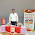Dear Bhanuchander, for Question no 11, the alphabets that can be overlapped are C,D,E,F,G,H,K,L,P - 9 letters only. B and R cannot be overlapped if observed carefully. (MISTRUST THE OBVIOUS)

1.while I agree that R might not be correct, but K is CORRECT since K in figure 1, assumes that it has gap between vertical line and the two diverging lines forming k, while in figure 2, there is no space between the vertical line and the other two slant lines.
I agree with you, but obvious and common sense sometimes out beats the so called intelligence!
Thank you sir for bringing this up.

2.Sir I m able to come up with 11 letters that can be overlapped B,C,D,E,F, G, H, J,L,P, R.PLZ TELL Where m I wrong?how Cum v r not considering j and r?

3.J is not possible since the curved u bend at the bottom is missing in the given figure (A). If you closely observe it, u can spot the diff.
Even R doesn't seem to overlap, it's hard to write it, put if u see the R alphabet given, assuming R is formed using three strokes - straight vertically up, curve, and then slant line, then from the transaction of second to third stroke, between the curve and the slant line, notice the gap between the slant line and the vertical straight line. This gap seems missing in the given overlapped image.

2.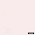Thanks bhanu sir...u r very helpful

3.Sir isn't there any mathematical concept which can be used in ques 12..?

1.i don't see any. If u come up with any, pl update.

2.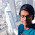Yes I think there is a method:-
1) The bigger gear can can spin 11 times so that the red arrow can come to its original position.
2) The smaller gear takes 8 turns to come back to its original position.
3) The last step is that when b completes 1 rotation the a gear will be 3 lesser that the original position do the some until they meet together.

4.Sir,for question 14 I dnt understand how we assume the cut out disc to be stationary

1.there's a concept in physics n maths called 'relative speed' in that if say two people move in opposite direction, 1km each per min, then in a min each would have moved 1 min ion the opposite direction, making the distance between them 2, this 2 km gap is called relative distrace, likewise for the disc system if the bottom is rotatin in one direction and the top part in reverse direction then for each unit of time, we need to add the amount moved by the both parts to get the relative distance between them,

5.Sir,For Q.no. 76 even C option is possible!(in the 1st row). tq

1.yes, agreed.

2.I believe even option A for last column last row and option D for second column last row

6.Sir can you please explain the 2nd question. Why should we count squares since we are asked to count rectangles.

1.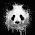Because a square is also a rectangle

7.Sir in q58 among all the 4 figs only in optionD,green is red on contact with yellow strip. In others it is white.It makes option D odd.please tell.

1.ya, in one way, what u said fits. Seems like the ques had several ans or we're not spotting their actual odd thing. Lets see for any other comments

8.Sir, In ques number 2 when its only asked for number of rectangles in the resultant figure then why are we adding up the number of squares?

1.square is a special kind of rectangle if i haven't mistaken!

9.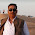ans no 13 is correctable ,how can i send correct answer figure ?

1.u can share it thru drive link so that even others can chk them. thank u

10.As per answer key in q22 I don't understand the use of printed booklet for tribes.can u plz explain how?

1.That's very obvious, since the women are from community with little exposure to the current. They would get news from word of mouth or through the other sources which is most likely hot them and those sources are news papers, TV, awareness campaign and distribution of booklets of even news paper with visuals or even explanations so that they would understand that.

2.But I believe considering them as tribes we can't expect them to read booklets?

11.for question no. 17 the official answer key gives the answer as 0.7-0.71

12.Sir can you explain question 79. ?

13.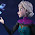Sir, for 16th question I got the same answer as you did, i.e, 0.625 . But according to the final answer key the answer is 0.7-0.71 . What should I do?

1.The are of the first disc is uneven so you need to take into consideration the area. I got this answer correct ��

2.You are right. I got the answer too. Thank you.

14.Sir, for question 17, the probability that 5 or 4 would come up on the first disc is more than two or three . Shouldn't we consider that?

1.well, yes, u have a valid point. Didn't thought about it. Will correct in free time. Thanks.

15.sir in question no. 2 why you have counted squares?

1.a rectangle might not fall under the category if square but a square can be considered as a form of rectangle. am i wrong?

16.Sir in Qno. 8 am to me answer is A,H,I,M,O,T,U,V,W,Y,X

1.i think u didn't understood the ques properly. The ques is when we flip the alphabets on horizontal axis, how many alphabets will still be alphabets in the same font. How can 'A' be possible. Try once again bro

17.Sir!for 11th question the alphabets that can be overlapped are BDEFHKLPR

18.bhanu sir i m board class and also preparing for uceed and nid ..plz help me to manage between my studys and nid and uceed prepration

1.putting aside the subjective part of NID, You can spare a minimum of 1 hr a day for both uceed or nid given that exams r near. Or you can chose to spend some hours in the weekend, like 4hrs on saturday n sunday. Also, make a priority list and try to cover them first so that u can be in safe side. For NID part B, regardless of ur sketch level, try to practice at least once in a week, attempting mostly on most relevant sketches, not going randomly. The better place is always start with prev. questions form NID official paper and then try most similar ques. When ur exams are around 10 days or a week, then increase the duration to min. 2-3hrs a day. U can chk the priority lists or one month practice list in this blog if u needed further help on topic selection.

19.bhanu sir good evening i have completed the numerical ques paper u have given but i found myself little bit weak in the question like'' A block of wood in the form of a cuboid 6cm × 4cm × 4cm has all its six faces painted pink. If the wooden block is cut into 96 cubes of 1cm × 1cm × 1cm, how many of these would have pink paint on them? ''
so how i cover these questions ..plz help me out sir

1.study non verbal topics. You can find such ques online also in any online apti or quiz websites. I think I've shared them already in uceed reasource page.

20.bhanu sir how one should be confident on his answer in NAT PART in UCEED

1.the moment u answered some ques, it mean u r confident of that. If not confident, spend little more time (within the set time) n if at least u get 70% positiveness, then go with that, else don't answer if it has negative marking.

21.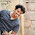Hello I have a doubt about question no 31 I don't think option C is a right answer because distance between 4 and 5 is not equal to distance between 5 and 6, so if option C was correct then there should have been a thin black line at the left end. Correct me if am wrong.

22.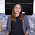Can someone please provide the correct solution for question number 16?

1.out of all the combinations, the combinations which add up to 7 or more are
(5,2), (5,3), (5,4), (5,5)
(4,3), (4,4), (4,5)
(3,4), (3,5)
(2,5)
And their respective probabilities will be 1/6x1/4 , 1/6x1/4, 1/3x1/4 and so on
So total probabity = Sum of all probabilities = 1/24+1/24+1/12+1/12+1/24+1/12+1/12+1/12+1/12+1/12= 17/24 = 0.708

2.How you have calculated the individual probabilities??? Pls. Help

23.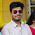Really helpful sir......I was searching for a website like this only it's really helpful . 😃

24.Can anyone tell , in Q11 why K is included in the answer . I think K would overlap fig.1 easily

25.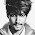Any resources for 21st type questions plz

1.plenty available. Have u ever tried resource page of this blog?

26.sir in Q3 just count the no of edges

1.can u give clear explanation ? no of edges means which part ?

2.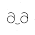Just counting the vertices will do as no. of vertices= no. of sides

3.Just count the no. of vertices. no. of vertices= no. of sides

27.Hello sir,

What is the logic behind Q34.

28.hello sir what is the logic for Q20

1.Since the corners of the cube are touching the hemisphere the length from the center to the opposite corners should be the radius of the hemisphere. Since the ladder is placed from the center to the opposite corner piece, it also has the same length as the radius, thus making the length of the ladder 10 m. Hope this helped.

29.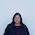please expalin q 63

30.please expalin Q 63

31.Sir in Q10 can the side of the fans blade also be considered as a circle when it rotates

32.Sir, Question 83, How do we know that black tin conducts the most heat?

33.In Ques No 58, I have a counter for B as in option D there is only one bright intersection and in all the other options there are equal number of bright and dark intersection patches(i.e 2)

34.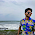In Question number 58,I have a counter for the option B as in option D there are unequal number of bright and dark patches at the intersection points(i.e 1&3) whereas in all the other options there are equal number of bright and dark patches(i.e 2&2)

35.doesn't q11 has a K in it?

36.are all the answers perfectly correct?

37.how to answer q34?

38.dear sir, for Q13 i can visualize 11 triangles according to the requirements.is it possible??

39.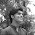Sir,in Q13 i can visualize 11 triangles according to the requirements of the question. Am I right?

40.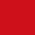I think reaching B is possible as well in Q. 82. I'm not being able to upload an image here, else I would have sent you and image of a possible path.

41.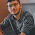42.Sir in question 13 there should be 11 triangles. One triangle is being made from the following circles: line 3 no.2 from right, line 6 no. 2 and 5 from right

43.Sir in question 13 there should be 11 triangles. One triangle is being made from the following circles: line 3 no.2 from right, line 6 no. 2 and 5 from right

44.Sir in question 13 there should be 11 triangles. One triangle is being made from the following circles: line 3 no.2 from right, line 6 no. 2 and 5 from right

45.Sir,why cant Q no.61 have A as its answer?i cannot differ between A and D.Whats the difference?

46.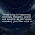sir, in question no. 30 i didnot get how option A comes as the answer.. could u pls show me if posiible?

47.sir, in question no.30 i didnt quite get how first option comes..maybe my lack of imagination but could u please show me again if possible?

48.In question 8
Those 11 letters According to question are: A H I M O T U V W X Y

49.In question number 12 why do we rotate gear A anticlacke

50.sir in que 76 option c is also right

51.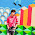Q 13 there are 11 triangles there s a huge triangle from right hand side corner.

52.can somebody pls explain ques 32 to me

53.Q3. count no. of corners
no. of corners =no.of sides

Your comment will be sent to Bhanu for his approval. It will get published after his approval. You will receive mail for all the replies to your comment.